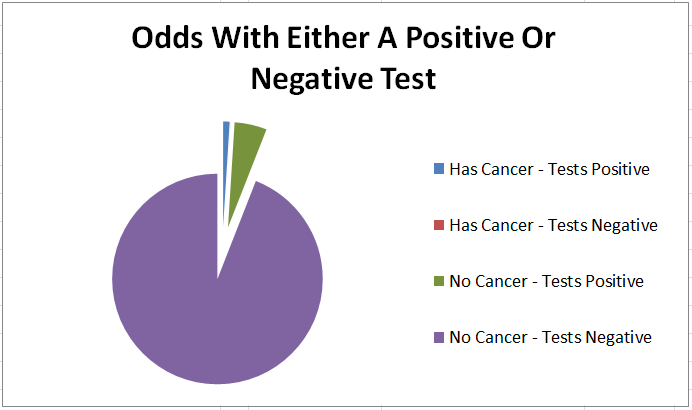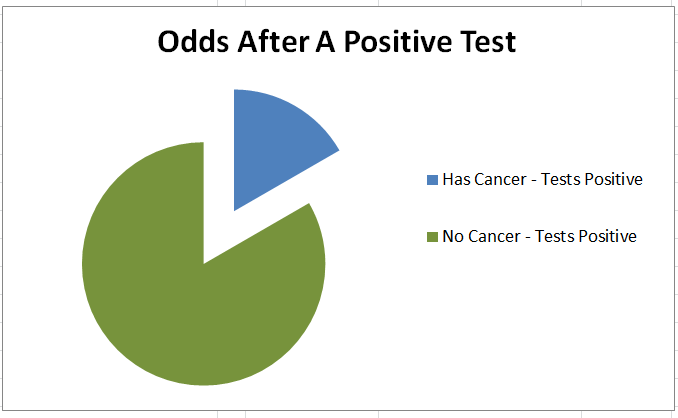# An Intuitive Guide To Bayes Theorem

The purpose of this page is to give you an intuitive understanding of how to solve Bayes Theorem problems.  The equation for Bayes Theorem is not all that clear, but Bayes Theorem itself is very intuitive.  The basics of Bayes Theorem are this

• Everything starts out with an initial probability – That is, before you do any tests or have any data, there is some initial probability of an event
• Tests can update that probability –  After you assign an initial probability, if you gather more information that is relevant then the probability can change.  For instance, you may initially have a very low chance of having an illness, but if a test for that illness comes back positive, the probability that you have it has increased
• After a test, all probabilities get normalized to 1 –  It doesn’t matter if an event is unlikely to have occurred.  What matters is if the event is likely compared to all other possible events.   For instance, if you don’t know whether you are observing a 6 sided die or a 20 sided die, and you see the die roll 4 five times in a row, it is unlikely that the 6 sided die would have rolled those values.  But it is extremely unlikely the 20 sided die would have, so comparatively the 6 sided die is more likely

## 6 Easy Steps For Any Bayes Problem

1. Determine what you want the probabilities for, and what you are observing
2. Estimate initial probabilities for all possible answers
3. For each of the initial possible answers, assume that it is true and calculate the probability of getting the observation with that possibility being true
4. Multiply the initial probabilities (Step 2) by the probabilities based on the observation (Step 3) for each of the initial possible answers
5. Normalize the results
6. Repeat steps 2-5 over and over for each new observation

## Bayes Theorem Applied To Cancer Testing

Testing for a disease is a classic Bayes Theorem problem, and one that can give counter intuitive results the first time you see it.   Let’s say that you are testing a generic patient for cancer.   One percent of the population has this cancer.   You have a test that will return a True Positive (return a positive when they actually do have cancer) 99% of the time, and return a True Negative (return a negative when they do not have cancer) 95% of the time.

You do 1 test, and get back a positive result.   What are the odds this patient actually does have this cancer ?

1. Determine Possibilities

There are two possibilities.  The patient either has cancer.  Or they do not have cancer

2. Estimate Initial Probabilities

Since  this is a generic patient they should be like the general population, so we assume there is a 1% chance they have cancer, and a 99% chance they do not.3. Calculate The Probability Of Getting The Result For Each Possible Answer

The result is a positive test.   If the patient has cancer, the probability of getting that result (True Positive) is 99%.   If the patient does not have cancer, the probability of getting that result (False Positive) is 5%  (which is 1 minus the 95% true negative rate)

4. Multiply Step 2 By Step 3 To Get The Combined Probability

This step should be similar to any other probability you have studied.  We are just calculating what is the probability they have cancer, and got a positive test.  And separately calculating what is the probability they do not have cancer, and got a positive test.5. Normalize The Results

This will be the final answer after 1 positive result.  At this step we see how likely the having cancer was, considering that a false positive was a possibilityAnd that is the answer, we have found that after the “99% Reliable” test, there is only a 16.7% chance that the patient has cancer

6. Repeat The Steps Over Again With Additional Observations

If you do additional tests, you use the new values as your starting probability.  In this case let’s assume that we do a second test, get a Positive result, and then a third test and get a Negative Result.

For the second test, the conditional equation is the same as the first test.  The normalized has cancer value of 16.7% gets multiplied by .99, and the normalized does not have cancer value of 83.33% gets multiplied by .05.

For the third test, since this was a negative result we need to change the formula.  We multiply the normalized has cancer probability by the False Negative rate of .01  (1-.99) , and the normalized does not have cancer rate by the True Negative rate of .95.

The results after both tests are shown below

##After the second positive result, the odds the patient actually has cancer jumps up to 79.8%, but after the negative test, the odds drop back down to 4%

## That Example Was Great, But You Promised Me Intuition

The page promised you intuition.  So far we have solved one Bayes Theorem problem, which is decent example, but not too different than what is on Wikipedia for Bayes Theorem.  Here is the intuition you should develop

• Bayes Theorem Is Just Multiplication and Division –  Bayes theorem itself is very simple.  Multiply out all of the strings of probabilities, and then normalize.  However some problems it is applied to are themselves very complicated, so the whole thing becomes complicated.  For instance, you can make the problem more difficult by using complicated probability distributions for the conditional probabilities or complicated initial probability distribution functions.  There might be special probability functions applied to a goal scoring problem in soccer, or a line waiting problem at a store.  But that doesn’t mean Bayes Theorem itself is all that complicated, the Bayes part of the problem is still just multiplication and division
• It is just as easy to solve for all possibilities as a single one – You might encounter a problem such as “This bag has 4 sided, 6 sided, 8 sided and 12 sided dice in it.  Your friend draws out a die, rolls it, and reports the number as 5.  What is the probability the selected die was a 6 sided die”  The problem asks you to solve for the 6 sided die, but since you have to get the total probability at each step any way in order to normalize, it is just as easy to solve the problem for the 4, 6, 8, and 12 sided dice at the same time.  Solving them all at the same time makes the thought process more straightforward, and can be done in a nice clean table.
• The order of observations doesn’t matter to end results – Bayes theorem amounts to repeated multiplication.  Multiplication is commutative.  You can change the order of the terms and get the same final results.   But if you change the order of the observations, for instance putting the negative cancer test result first in our example problem, the intermediate results will have different probabilities
• You Don’t Actually Have To Normalize Each Step – We normalized the example problem each step.  You could do all of the multiplication for the observations, and then normalize at the end and get the same result.  The only caution is that the probabilities can get very small after repeated decimal multiplication if you do not normalize. You can run into trouble with round off or truncation error depending on what you are using to do the math.

## So Why Was The Cancer Problem Result Surprising ?

Many people are surprised to see that a positive result on the 99% reliable test still only means there was a 16.7% chance the patient had cancer.  Why was that surprising ?  Because most people do not bake the initial probabilities into their intuition.

We do a good job of understanding the conditional probability.  After all, a 99% reliable test should make it much more likely the patient has cancer, which it does.  But if the initial probability is a really small number, the new probability will probably be small as well.  This often gets overlooked, and people implicitly assume an evenly distributed initial probability when thinking about these types of problems.

Overlooking the initial probability is the real joke behind this XKCD comic https://xkcd.com/1132/   (not having to pay the bet if the sun actually exploded is merely a bonus)

These pie charts are a good way to visualize what is occurring.  If the patient doesn’t do any test, the odds of having cancer are a small slice of the pieWhile the patient is waiting for the test results there are 4 possibilities, either they have cancer and the test comes back positive (blue),  they don’t have cancer and the test comes back positive (green),  they don’t have cancer and the test comes back negative (purple)  or they have cancer and the test comes back negative (red slice, but too small to be seen)Once they get positive test results, the purple and red slices of the previous chart go away.   We normalize the green and blue slices in light of the new total probability. The odds of getting that result due to a false positive (green) are still larger than the odds of a true positive (blue).## More Examples

If you want more examples and information about Bayes Theorem, here is a book I wrote walking through half a dozen Bayes Theorem examplesAnd here is an Excel file solution to some Bayes Theorem problems